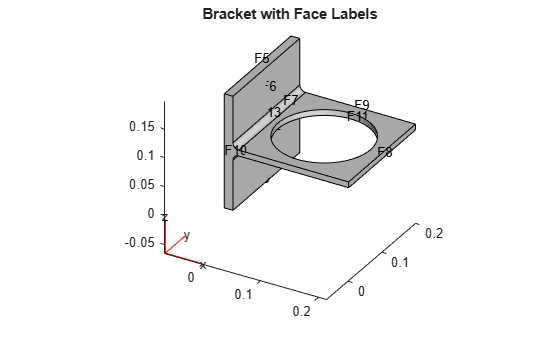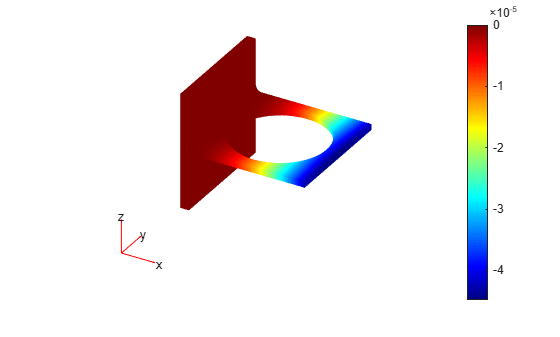# StationaryResults

Time-independent PDE solution and derived quantities

## Description

A `StationaryResults` object contains the solution of a PDE and its gradients in a form convenient for plotting and postprocessing.

• A `StationaryResults` object contains the solution and its gradient calculated at the nodes of the triangular or tetrahedral mesh, generated by `generateMesh`.

• Solution values at the nodes appear in the `NodalSolution` property.

• The three components of the gradient of the solution values at the nodes appear in the `XGradients`, `YGradients`, and `ZGradients` properties.

• The array dimensions of `NodalSolution`, `XGradients`, `YGradients`, and `ZGradients` enable you to extract solution and gradient values for specified equation indices in a PDE system.

To interpolate the solution or its gradient to a custom grid (for example, specified by `meshgrid`), use `interpolateSolution` or `evaluateGradient`.

## Creation

There are several ways to create a `StationaryResults` object:

• Solve a time-independent problem using the `solvepde` function. This function returns a PDE solution as a `StationaryResults` object. This is the recommended approach.

• Solve a time-independent problem using the `assempde` or `pdenonlin` function. Then use the `createPDEResults` function to obtain a `StationaryResults` object from a PDE solution returned by `assempde` or `pdenonlin`. Note that `assempde` and `pdenonlin` are legacy functions. They are not recommended for solving PDE problems.

## Properties

expand all

Finite element mesh, returned as a FEMesh Properties object.

Solution values at the nodes, returned as a vector or array. For details about the dimensions of `NodalSolution`, see Dimensions of Solutions, Gradients, and Fluxes.

Data Types: `double`

x-component of the gradient at the nodes, returned as a vector or array. For details about the dimensions of `XGradients`, see Dimensions of Solutions, Gradients, and Fluxes.

Data Types: `double`

y-component of the gradient at the nodes, returned as a vector or array. For details about the dimensions of `YGradients`, see Dimensions of Solutions, Gradients, and Fluxes.

Data Types: `double`

z-component of the gradient at the nodes, returned as a vector or array. For details about the dimensions of `ZGradients`, see Dimensions of Solutions, Gradients, and Fluxes.

Data Types: `double`

## Object Functions

 `evaluateCGradient` Evaluate flux of PDE solution `evaluateGradient` Evaluate gradients of PDE solutions at arbitrary points `interpolateSolution` Interpolate PDE solution to arbitrary points

## Examples

collapse all

Create a PDE model for a system of three equations. Import the geometry of a bracket and plot the face labels.

```model = createpde(3); importGeometry(model,"BracketWithHole.stl"); figure pdegplot(model,"FaceLabels","on") view(30,30) title("Bracket with Face Labels")``````figure pdegplot(model,"FaceLabels","on") view(-134,-32) title("Bracket with Face Labels, Rear View")```Set boundary conditions such that face 4 is immobile, and face 8 has a force in the negative `z` direction.

```applyBoundaryCondition(model,"dirichlet","Face",4,"u",[0,0,0]); applyBoundaryCondition(model,"neumann","Face",8,"g",[0,0,-1e4]);```

Set coefficients that represent the equations of linear elasticity. See Linear Elasticity Equations.

```E = 200e9; nu = 0.3; specifyCoefficients(model,"m",0,... "d",0,... "c",elasticityC3D(E,nu),... "a",0,... "f",[0;0;0]);```

Create a mesh.

`generateMesh(model,"Hmax",1e-2);`

Solve the PDE.

`results = solvepde(model)`
```results = StationaryResults with properties: NodalSolution: [14314x3 double] XGradients: [14314x3 double] YGradients: [14314x3 double] ZGradients: [14314x3 double] Mesh: [1x1 FEMesh] ```

Access the solution at the nodal locations.

`u = results.NodalSolution;`

Plot the solution for the `z`-component, which is component 3.

`pdeplot3D(model,"ColorMapData",u(:,3))`Obtain a `StationaryResults` object from a legacy solver together with `createPDEResults`.

Create a PDE model for a system of three equations. Import the geometry of a bracket and plot the face labels.

```model = createpde(3); importGeometry(model,"BracketWithHole.stl"); figure pdegplot(model,"FaceLabels","on") view(30,30) title("Bracket with Face Labels")``````figure pdegplot(model,"FaceLabels","on") view(-134,-32) title("Bracket with Face Labels, Rear View")```Set boundary conditions such that `F4` is immobile, and `F8` has a force in the negative `z` direction.

```applyBoundaryCondition(model,"dirichlet","Face",4,"u",[0,0,0]); applyBoundaryCondition(model,"neumann","Face",8,"g",[0,0,-1e4]);```

Set coefficients for a legacy solver that represent the equations of linear elasticity. See Linear Elasticity Equations.

```E = 200e9; nu = 0.3; c = elasticityC3D(E,nu); a = 0; f = [0;0;0];```

Create a mesh.

`generateMesh(model,"Hmax",1e-2);`

Solve the problem using a legacy solver.

`u = assempde(model,c,a,f);`

Create a `StationaryResults` object from the solution.

`results = createPDEResults(model,u)`
```results = StationaryResults with properties: NodalSolution: [14314x3 double] XGradients: [14314x3 double] YGradients: [14314x3 double] ZGradients: [14314x3 double] Mesh: [1x1 FEMesh] ```

Access the solution at the nodal locations.

`u = results.NodalSolution;`

Plot the solution for the `z`-component, which is component 3.

`pdeplot3D(model,"ColorMapData",u(:,3))`## Version History

Introduced in R2016a

expand all